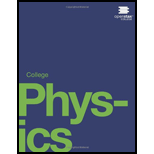# The total energy in the beam of an accelerator is far greater than the energy of the individual beam particles. Why isn't this total energy available to create a single extremely massive particle?### College Physics

1st Edition
Paul Peter Urone + 1 other
Publisher: OpenStax College
ISBN: 9781938168000### College Physics

1st Edition
Paul Peter Urone + 1 other
Publisher: OpenStax College
ISBN: 9781938168000

#### Solutions

Chapter
Section
Chapter 33, Problem 1CQ
Textbook Problem

## The total energy in the beam of an accelerator is far greater than the energy of the individual beam particles. Why isn't this total energy available to create a single extremely massive particle?

Expert Solution
To determine

Why isn't total energy available to create a single extremely massive particle?

Einstein has stated in his theory that any particle having mass cannot travel more than the speed of light without its mass becoming infinite. The particle accelerator accelerates the particle close to speed of light.

### Explanation of Solution

The energy supplied to the particle is given by the formula:

E=mc2

Here, m is the mass of particle and c is the speed of particle. So to increase the speed of light the particle mass should be decreased for constant energy.

Einstein has stated in his theory that any particle having mass cannot travel more than the speed of light without its mass becoming infinite. The particle accelerator accelerates the particle close to speed of light.

Since the energy supplied is finite, to increase the speed of the particle its mass should be small. Therefore, a beam of particles having very small individual mass are accelerated in the particle accelerator.

Conclusion:

The energy supplied to the particle is given by the formula:

E=mc2

Here, m is the mass of particle and c is the speed of particle. So to increase the speed of light the particle mass should be decreased for constant energy.

### Want to see more full solutions like this?

Subscribe now to access step-by-step solutions to millions of textbook problems written by subject matter experts!

Get Solutions

### Want to see more full solutions like this?

Subscribe now to access step-by-step solutions to millions of textbook problems written by subject matter experts!

Get Solutions